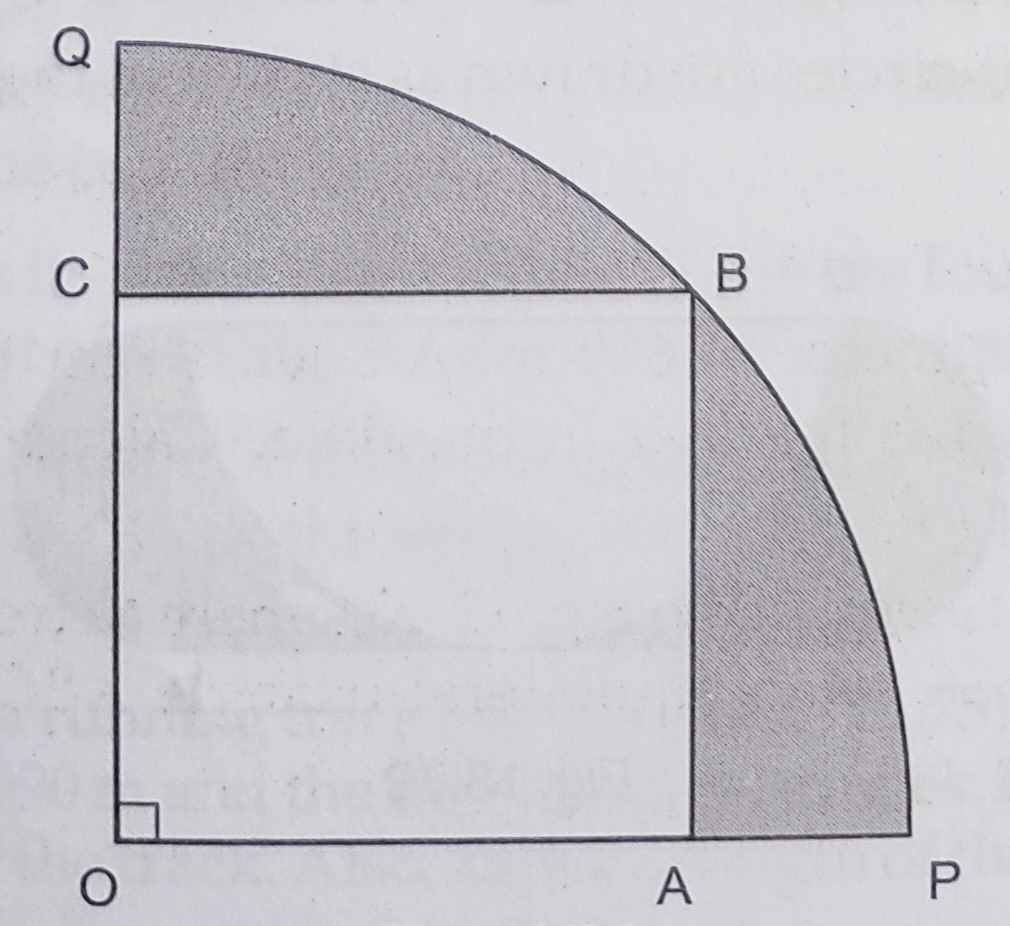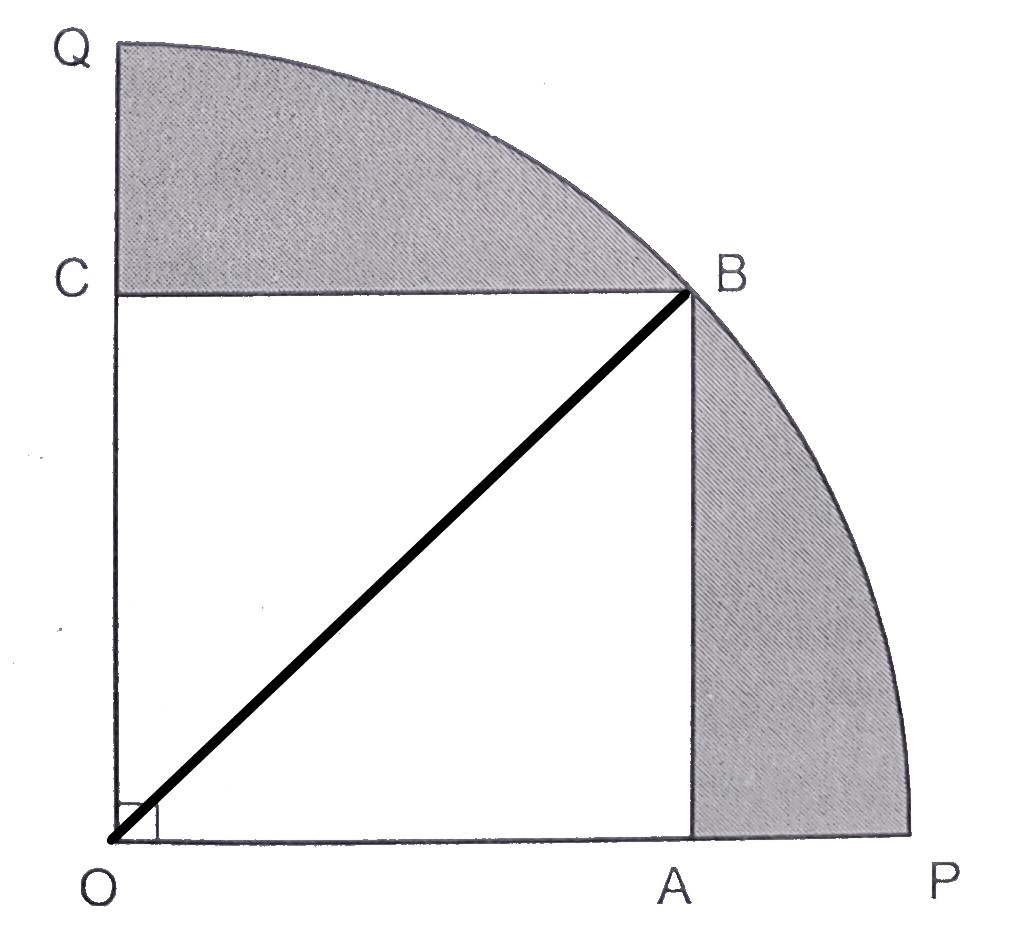"
">

# In the below figure, a square $O A B C$ is inscribed in a quadrant $O P B Q$ of a circle. If $O A=21 \mathrm{~cm}$, find the area of the shaded region."

Given:

A square $O A B C$ is inscribed in a quadrant $O P B Q$ of a circle.

$O A=21 \mathrm{~cm}$.

To do:

We have to find the area of the shaded region.

Solution:

From the figure,
$OABC$ is a square.

$OA = 21\ cm$Join $OB$,

This implies,

Diagonal of the square $\mathrm{OB}=\sqrt{2} \times \mathrm{OA}$

$=\sqrt{2} \times 21 \mathrm{~cm}$

$=21 \sqrt{2} \mathrm{~cm}$

Radius of the quadrant $=21 \sqrt{2} \mathrm{~cm}$

Therefore,

Area of the shaded region $=$ Area of quadrant $-$ Area of square

$=\frac{1}{4} \pi r^{2}-(\mathrm{OA})^{2}$

$=\frac{1}{4} \times \frac{22}{7} \times(21 \sqrt{2})^{2}-(21)^{2}$

$=\frac{11}{14} \times 441 \times 2-441$

$=441(\frac{22}{14}-1)$

$=441 \times \frac{8}{14}$

$=252 \mathrm{~cm}^{2}$

The area of the shaded region is $252\ cm^2$.

Updated on: 10-Oct-2022

33 Views